# Compress Neural Network Using Projection

This example shows how to compress a neural network using projection and principal component analysis.

To compress a deep learning network, you can use projected layers. The layer introduces learnable projector matrices $Q$, replaces multiplications of the form $Wx$, where $W$ is a learnable matrix, with the multiplication $WQ{Q}^{\top }x$, and stores $Q$ and $W\prime =WQ$ instead of storing $W$. In some cases, this is equivalent to replacing a layer with a subnetwork of smaller layers. Projecting $x$ into a lower dimensional space using $Q$ typically requires less memory to store the learnable parameters and can have similarly strong prediction accuracy. A projected deep neural network can also exhibit faster forward passes when run on the CPU or deployed to embedded hardware using library-free C or C++ code generation.

The `compressNetworkUsingProjection` function compresses a network by projecting layers into smaller parameter subspaces. For optimal initialization of the projected network, the function projects the learnable parameters of projectable layers into a subspace that maintains the highest variance in neuron activations. After you compress a neural network using projection, you can then fine-tune the network to increase the accuracy.

This chart shows the effect of projection and fine tuning on a trained network. In this case, the projected network has significantly fewer learnable parameters at the cost of classification accuracy. The fine-tuned projected network yields similar classification accuracy to the original network.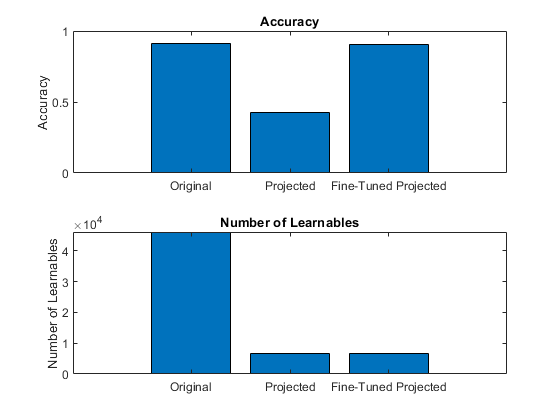Load the pretrained network in `dlnetJapaneseVowels`.

`load dlnetJapaneseVowels`

View the network layers. The network is a LSTM network with a single LSTM layer with 100 hidden units.

`net.Layers`
```ans = 4×1 Layer array with layers: 1 'sequenceinput' Sequence Input Sequence input with 12 dimensions 2 'lstm' LSTM LSTM with 100 hidden units 3 'fc' Fully Connected 9 fully connected layer 4 'softmax' Softmax softmax ```

View the class names.

`classNames`
```classNames = 9×1 string "1" "2" "3" "4" "5" "6" "7" "8" "9" ```

This example trains several networks. For comparison, create a copy of the original network.

`netOriginal = net;`

Load the Japanese Vowels data set described in  and  that contains 270 sequences of varying length with 12 features corresponding to LPC cepstrum coefficients and a categorical vector of labels 1, 2, ..., 9. The sequences are matrices with 12 rows (one row for each feature) and a varying number of columns (one column for each time step).

`load JapaneseVowelsTrainData`

### Analyze Neuron Activations for Compression Using Projection

The `compressNetworkUsingProjection` function uses principal component analysis (PCA) to identify the subspace of learnable parameters that result in the highest variance in neuron activations by analyzing the network activations using a data set of training data. This analysis requires only the predictors of the training data to compute the network activations. It does not require the training targets.

The PCA step can be computationally intensive. If you expect to compress the same network multiple times (for example, when exploring different levels of compression), then perform the PCA step first and reuse the resulting `neuronPCA` object.

Create a mini-batch queue containing the training data. To create a mini-batch queue from in-memory data, convert the sequences to an array datastore.

`adsXTrain = arrayDatastore(XTrain,OutputType="same");`

Create the `minibatchqueue` object.

• Specify a mini-batch size of 16.

• Preprocess the mini-batches using the `preprocessMiniBatchPredictors` function, listed in the Mini-Batch Predictors Preprocessing Function section of the example.

• Specify that the output data has format `"CTB"` (channel, time, batch).

Note: Do not pad sequence data when doing the PCA step for projection as this can negatively impact the analysis. Instead, truncate mini-batches of data to have the same length or use mini-batches of size 1.

```miniBatchSize = 16; mbqTrain = minibatchqueue(adsXTrain, ... MiniBatchSize=miniBatchSize, ... MiniBatchFcn=@preprocessMiniBatchPredictors, ... MiniBatchFormat="CTB");```

Create the `neuronPCA` object. To view information about the steps of the neuron PCA algorithm, set the `VerbosityLevel` option to `"steps"`.

`npca = neuronPCA(netOriginal,mbqTrain,VerbosityLevel="steps");`
```Using solver mode "direct". Computing covariance matrices for activations connected to: "lstm/in","lstm/out","fc/in","fc/out" Computing eigenvalues and eigenvectors for activations connected to: "lstm/in","lstm/out","fc/in","fc/out" neuronPCA analyzed 2 layers: "lstm","fc" ```

View the properties of the `neuronPCA` object.

`npca`
```npca = neuronPCA with properties: LayerNames: ["lstm" "fc"] ExplainedVarianceRange: [0 1] LearnablesReductionRange: [0 0.9690] InputEigenvalues: {[12×1 double] [100×1 double]} InputEigenvectors: {[12×12 double] [100×100 double]} OutputEigenvalues: {[100×1 double] [9×1 double]} OutputEigenvectors: {[100×100 double] [9×9 double]} ```

### Project Network

Compress the network using the neuron PCA object.

`netProjected = compressNetworkUsingProjection(netOriginal,npca);`
```Compressed network has 83.4% fewer learnable parameters. Projection compressed 2 layers: "lstm","fc" ```

### Test Projected Network

Load the Japanese Vowels test data set.

`load JapaneseVowelsTestData`

Create a mini-batch queue using the same steps as the training data.

```adsTest = arrayDatastore(XTest,OutputType="same"); mbqTest = minibatchqueue(adsTest, ... MiniBatchSize=miniBatchSize, ... MiniBatchFcn=@preprocessMiniBatchPredictors, ... MiniBatchFormat="CTB");```

For comparison, calculate the classification accuracy of the original network using the test data and the `modelPredictions` function, listed in the Model Predictions Function section of the example.

```YTest = modelPredictions(netOriginal,mbqTest,classNames); accOriginal = mean(YTest == TTest)```
```accOriginal = 0.9135 ```

Calculate the classification accuracy of the projected network using the test data and the `modelPredictions` function, listed in the Model Predictions Function section of the example.

```YTest = modelPredictions(netProjected,mbqTest,classNames); accProjected = mean(YTest == TTest)```
```accProjected = 0.4811 ```

Compare the accuracy and the number of learnables of each network in a bar chart. To calculate the number of learnables of each network, use the `numLearnables` function, listed in the Number of Learnables Function section of the example.

```figure tiledlayout("flow") nexttile bar([accOriginal accProjected]) xticklabels(["Original" "Projected"]) title("Accuracy") ylabel("Accuracy") nexttile bar([numLearnables(netOriginal) numLearnables(netProjected)]) xticklabels(["Original" "Projected"]) ylabel("Number of Learnables") title("Number of Learnables")```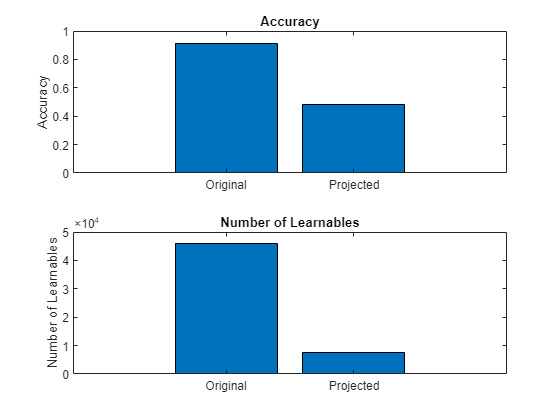The projected network yields worse classification accuracy and has significantly fewer learnable parameters.

### Compress for Memory Requirement

If you want to compress a network so that it meets specific hardware memory requirements, then you can manually calculate the learnable reduction value such that the compressed network is of the desired size.

Specify a target memory requirement of 64 kilobytes ($64×1024$ bytes).

`targetMemorySize = 64*1024`
```targetMemorySize = 65536 ```

Calculate the memory size of the original network using the `parameterMemory` function, listed in the Parameter Memory Function section of the example.

`memorySizeOriginal = parameterMemory(netOriginal)`
```memorySizeOriginal = 184436 ```

Calculate the factor to reduce the learnables by such that the resulting network meets the memory requirements.

`reductionGoal = 1 - (targetMemorySize/memorySizeOriginal);`

Project the network using the `compressNetworkUsingProjection` function and set the `LearnablesReductionGoal` option to the calculated reduction factor.

```netProjected = compressNetworkUsingProjection(netOriginal,npca, ... LearnablesReductionGoal=reductionGoal);```
```Compressed network has 64.8% fewer learnable parameters. Projection compressed 2 layers: "lstm","fc" ```

Calculate the memory size of the projected network using the `parameterMemory` function, listed in the Parameter Memory Function section of the example.

`memorySizeProjected = parameterMemory(netProjected)`
```memorySizeProjected = 64844 ```

Calculate the classification accuracy of the projected network using the test data and the `modelPredictions` function, listed in the Model Predictions Function section of the example.

```YTest = modelPredictions(netProjected,mbqTest,classNames); accProjected = mean(YTest == TTest)```
```accProjected = 0.8595 ```

Compare the accuracy and the memory size of each network in a bar chart.

```figure tiledlayout("flow") nexttile bar([accOriginal accProjected]) xticklabels(["Original" "Projected"]) ylabel("Accuracy") title("Accuracy") nexttile bar([memorySizeOriginal memorySizeProjected]) xticklabels(["Original" "Projected"]) yline(targetMemorySize,"r--","Memory Requirement") ylabel("Memory (bytes)") title("Memory Size")```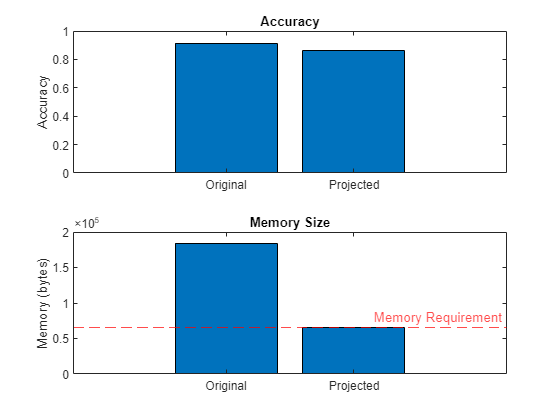The projected network yields similar classification accuracy and has memory size that meets the memory requirements.

### Explore Compression Levels

There is a trade-off between the amount of compression and the network accuracy. In particular, reducing the number of learnable parameters typically reduces the network accuracy.

The explained variance of a network details how well the space of network activations can capture the underlying features of the data. To explore different amounts of compression, you can iterate over different values of the `ExplainedVarianceGoal` option of the `compressNetworkUsingProjection` function and compare the results.

Loop over different values of the explained variance goal. Iterate over 20 logarithmically spaced values between 0.999 and 0.

For each value:

• Compress the network using projection with the specified explained variance goal using the `compressNetworkUsingProjection` function. Suppress verbose output by setting the `VerbosityLevel` option to `"off"`.

• Record the actual explained variance and learnables reduction of the projected network.

• Calculate the classification accuracy of the projected network using the test data and the `modelPredictions` function, listed in the Model Predictions Function section of the example.

```numValues = 20; explainedVarGoal = 1 - logspace(-3,0,numValues); for i = 1:numel(explainedVarGoal) varianceGoal = explainedVarGoal(i); [netProjected,info] = compressNetworkUsingProjection(netOriginal,npca, ... ExplainedVarianceGoal=varianceGoal, ... VerbosityLevel="off"); explainedVariance(i) = info.ExplainedVariance; learnablesReduction(i) = info.LearnablesReduction; YTest = modelPredictions(netProjected,mbqTest,classNames); accuracy(i) = mean(YTest==TTest); end```

Visualize the effect of the different settings of the explained variance goal in a plot.

```figure tiledlayout("flow") nexttile plot(learnablesReduction,accuracy,'+-') ylabel("Accuracy") title("Effect of Explained Variance Goal") nexttile plot(learnablesReduction,explainedVariance,'+-') ylim([0 inf]) ylabel("Explained Variance") xlabel("Learnable Reduction")```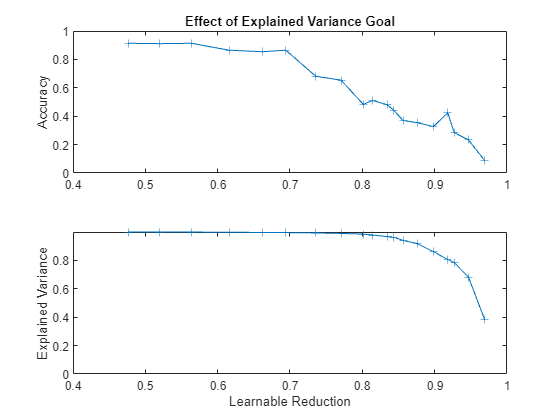The graphs show that an increase in learnable reduction has a corresponding decrease in the explained variance and accuracy. A learnable reduction value of around 85% shows a very slight decrease in explained variance and a small decrease in accuracy.

Compress the network using projection with a learnable reduction goal of 85% using the `compressNetworkUsingProjection` function. Suppress verbose output by setting the `VerbosityLevel` option to `"off"`.

```netProjected = compressNetworkUsingProjection(netOriginal,npca, ... LearnablesReduction=0.85, ... VerbosityLevel="off");```

Calculate the classification accuracy of the projected network using the test data and the `modelPredictions` function, listed in the Model Predictions Function section of the example.

```YTest = modelPredictions(netProjected,mbqTest,classNames); accProjected = mean(YTest == TTest)```
```accProjected = 0.4351 ```

Compare the accuracy and the number of learnables of each network in a bar chart. To calculate the number of learnables of each network, use the `numLearnables` function, listed in the Number of Learnables Function section of the example.

```figure tiledlayout("flow") nexttile bar([accOriginal accProjected]) xticklabels(["Original" "Projected"]) ylabel("Accuracy") title("Accuracy") nexttile bar([numLearnables(netOriginal) numLearnables(netProjected)]) xticklabels(["Original" "Projected"]) ylabel("Number of Learnables") title("Number of Learnables")```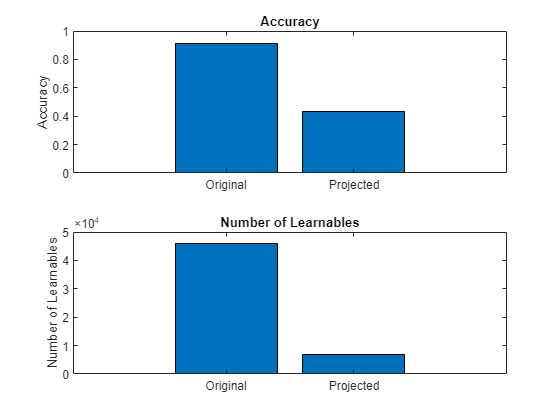The projected network yields worse classification accuracy and has significantly fewer learnable parameters. You can improve the network accuracy by fine tuning the network.

### Fine-Tune Compressed Network

Compressing a network using projection typically reduces the network accuracy. To improve the accuracy, fine-tune (retrain) the network.

Specify the training options. Choosing among the options requires empirical analysis. To explore different training option configurations by running experiments, you can use the Experiment Manager app.

• Because the input sequences have rows and columns that correspond to channels and time steps, respectively, specify input data formats of `"CTB"` (channel, time, batch).

• Train with a mini-batch size of 16.

• For fine-tuning, lower the learning rate to 0.0005.

• Shuffle the data every epoch.

• Monitor the training progress in a plot and monitor the accuracy metric.

• Disable the verbose output.

```options = trainingOptions("adam", ... InputDataFormats="CTB", ... MiniBatchSize=16, ... InitialLearnRate=0.0005, ... Shuffle="every-epoch", ... Plots="training-progress", ... Metrics="accuracy", ... Verbose=false);```

Train the neural network using the `trainnet` function. For classification, use cross-entropy loss. By default, the `trainnet` function uses a GPU if one is available. Training on a GPU requires a Parallel Computing Toolbox™ license and a supported GPU device. For information on supported devices, see GPU Computing Requirements (Parallel Computing Toolbox). Otherwise, the trainnet function uses the CPU. To specify the execution environment, use the `ExecutionEnvironment` training option.

`netFineTuned = trainnet(XTrain,TTrain,netProjected,"crossentropy",options);`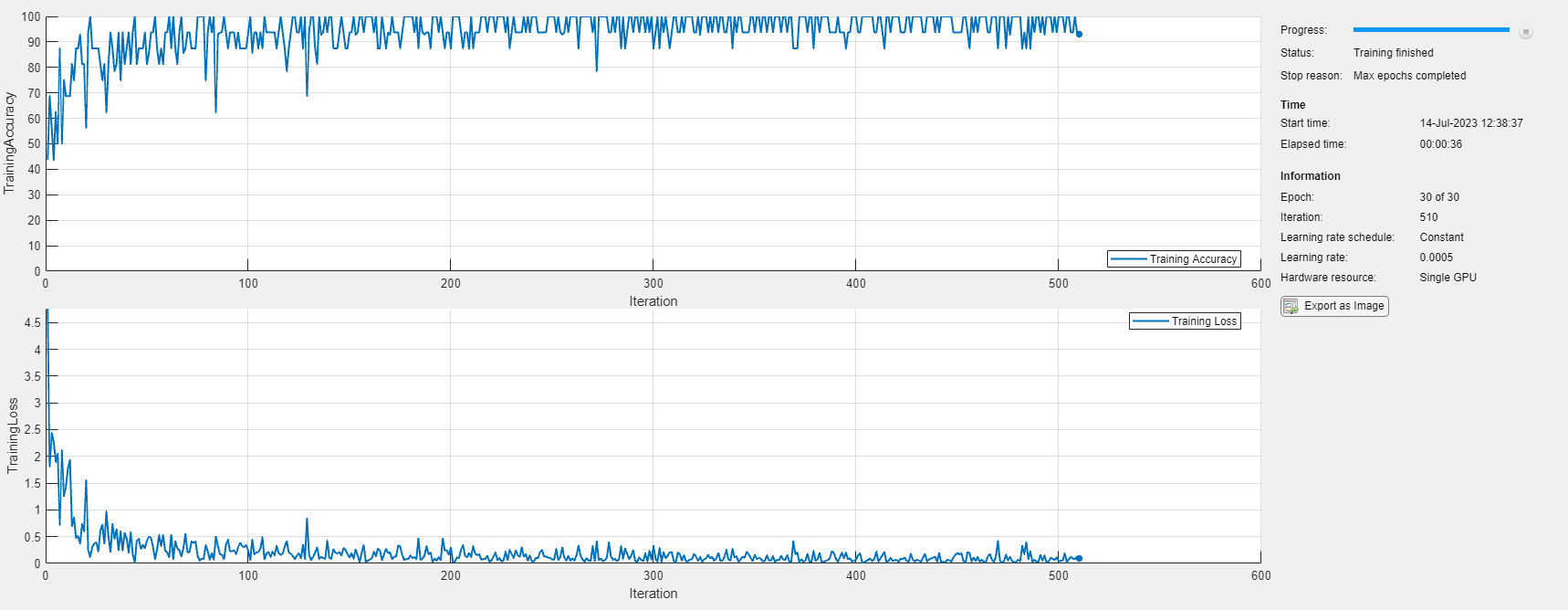### Test Fine-Tuned Network

Calculate the classification accuracy of the fine-tuned network using the test data and the `modelPredictions` function, listed in the Model Predictions Function section of the example.

```YTest = modelPredictions(netFineTuned,mbqTest,classNames); accFineTuned = mean(YTest == TTest)```
```accFineTuned = 0.8622 ```

Compare the accuracy and the number of learnables of each network in a bar chart. To calculate the number of learnables of each network, use the `numLearnables` function, listed in the Number of Learnables Function section of the example.

```figure tiledlayout("flow") nexttile bar([accOriginal accProjected accFineTuned]) xticklabels(["Original" "Projected" "Fine-Tuned Projected"]) title("Accuracy") ylabel("Accuracy") nexttile bar([numLearnables(netOriginal) numLearnables(netProjected) numLearnables(netFineTuned)]) xticklabels(["Original" "Projected" "Fine-Tuned Projected"]) ylabel("Number of Learnables") title("Number of Learnables")```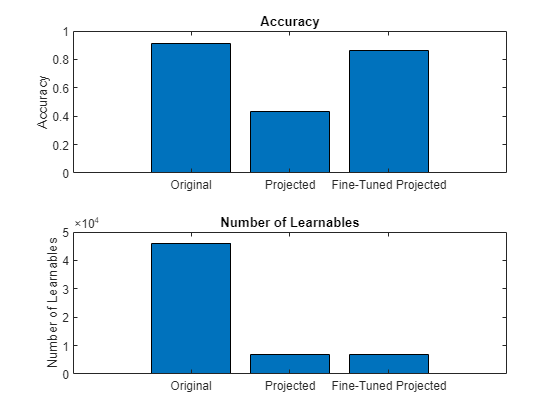The projected network has significantly fewer learnable parameters at the cost of classification accuracy. The fine-tuned projected network yields similar classification accuracy to the original network.

### Supporting Functions

#### Mini-Batch Predictors Preprocessing Function

The `preprocessMiniBatchPredictors` function preprocesses a mini-batch of predictors by extracting the sequence data from the input cell array and truncating them along the second dimension so that they have the same length.

Note: Do not pad sequence data when doing the PCA step for projection as this can negatively impact the analysis. Instead, truncate mini-batches of data to have the same length or use mini-batches of size 1.

```function X = preprocessMiniBatchPredictors(dataX) X = padsequences(dataX,2,Length="shortest"); end```

#### Number of Learnables Function

The `numLearnables` function returns the total number of learnables in a network.

```function N = numLearnables(net) N = 0; for i = 1:size(net.Learnables,1) N = N + numel(net.Learnables.Value{i}); end end```

#### Parameter Memory Function

The `parameterMemory` function returns the size in bytes of the learnable parameters of a network, where the learnable parameters are in single precision (4 bytes per learnable).

```function numBytes = parameterMemory(net) numBytes = 4*numLearnables(net); end```

#### Model Predictions Function

The `modelPredictions` function takes a `dlnetwork` object `net`, a `minibatchqueue` of input data `mbq`, and the network classes, and computes the model predictions by iterating over all data in the `minibatchqueue` object. The function uses the `onehotdecode` function to find the predicted class with the highest score.

```function Y = modelPredictions(net,mbq,classNames) Y = []; reset(mbq) while hasdata(mbq) X = next(mbq); scores = predict(net,X); labels = onehotdecode(scores,classNames,1)'; Y = [Y; labels]; end end```

### Bibliography

1. M. Kudo, J. Toyama, and M. Shimbo. "Multidimensional Curve Classification Using Passing-Through Regions." Pattern Recognition Letters. Vol. 20, No. 11–13, pages 1103–1111.

2. UCI Machine Learning Repository: Japanese Vowels Dataset. https://archive.ics.uci.edu/ml/datasets/Japanese+Vowels